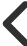Ideas Post### Maximillian Hill

@mkhill1212# 10 Formulas Every Business Owner Should Know

## 1. Inventory Turnover = Cost of Goods Sold/Average Inventory

Indicates the amount of money tied up in inventory, and thus inventory management effectiveness. The higher the turnover ratio, the better.

## 2. Receivables Turnover = Revenue/ Average Receivables

A high receivables turnover could mean either that collection is efficient, or that credit policies are too stringent.

## 3. Payables Turnover = Purchases/ Average Trade Payables

Cost of Goods Sold is often used as an approximation of purchases

## 4. Current Ratio = Current Assets/Current Liabilities

"Current Assets" means assets expected to be consumed or converted to cash within one year. A lower ratio means greater reliance on operating cash flow and financing to meet short term obligations.

## 5. Quick Ratio = Cash + Short Term Marketable Investments + Receivables/Current Liabilities

Includes only the more liquid "current" assets. This ratio is more conservative than the current ratio.

## 6. Cash conversion cycle = Days on hand + Days sales outstanding - # Days payable

The amount of time between the company investing in working capital, and collecting cash. A short cash-conversion cycle indicates greater liquidity.

## 7. Debt to Assets Ratio = Total Debt/Total Assets

The percentage of total assets financed by debt. Higher debt means higher financial risk and weaker solvency.

## 8. Debt to Equity Ratio = Total Debt/Total Shareholder's Equity

The amount of debt capital relative to equity capital. A ratio of 1 indicates equal amounts of debt and equity.

## 9. Gross Profit Margin = Gross Profit/Revenue

A high gross profit margin is the result of low materials cost and/or high product pricing.

## 10. Operating Profit Margin = Operating Income/Revenue

Changes in this ratio can reflect improvements or deterioration in operating costs, such as overhead.

0 Like.0 CommentMarkand 5 more liked this023960800